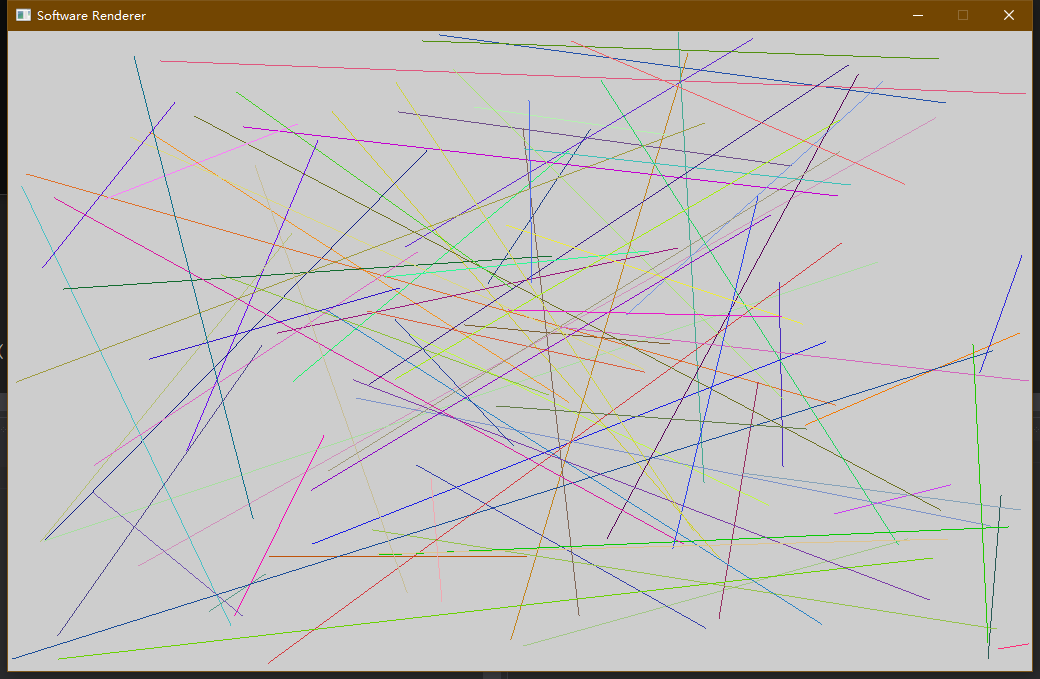## 代码部分：

``````void Canvas::DrawLine(Color& c, int x0, int y0, int x1, int y1)
{
if (x0 == x1 && y0 == y1)
{
DrawPixel(c, x0, y0);
}
else if (x0 == x1)
{
// increment direction
int inc = (y0 < y1) ? 1 : -1;
for (int y = y0; y != y1; y += inc)
{
DrawPixel(c, x0, y);
}
DrawPixel(c, x1, y1);
}
else if (y0 == y1)
{
// increment direction
int inc = (x0 < x1) ? 1 : -1;
for (int x = x0; x != x1; x += inc)
{
DrawPixel(c, x, y0);
}
DrawPixel(c, x1, y1);
}
else
{
int dx = abs(x1 - x0);
int dy = abs(y1 - y0);

// -1 <= Slope <= 1
if (dx >= dy)
{
// Accumulation
int acc = 0;
// increment direction
int incX = (x0 > x1) ? -1 : 1;
int incY = (y0 > y1) ? -1 : 1;
for (int x = x0, y = y0; x != x1 && y != y1; x += incX)
{
DrawPixel(c, x, y);
// Accumulate short side
acc += dy;
if (acc >= dx)
{
acc -= dx;
y += incY;
}
}
DrawPixel(c, x1, y1);
}
// Slope > 1 || Slope < -1
else
{
// Accumulation
int acc = 0;
// increment direction
int incX = (x0 > x1) ? -1 : 1;
int incY = (y0 > y1) ? -1 : 1;
for (int x = x0, y = y0; x != x1 && y != y1; y += incY)
{
DrawPixel(c, x, y);
// Accumulate short side
acc += dx;
if (acc >= dy)
{
acc -= dy;
x += incX;
}
}
DrawPixel(c, x1, y1);
}
}
}
``````

``````			for (int x = x0, y = y0; x != x1 && y != y1; y += incY)
{
DrawPixel(c, x, y);
// Accumulate short side
acc += dx;
if (acc >= dy)
{
acc -= dy;
x += incX;
}
}
``````

``````int main(int argc, char* args[])
{
if (!init())
{
printf("Failed to initialize!\n");
}

else
{
// Rendering loop
bool bQuit = false;
while (!bQuit)
{
SDL_Event event;
while (SDL_PollEvent(&event))
{
if (event.type == SDL_QUIT)
{
bQuit = true;
}
}

Color c(Random::GetUint32());
cav->DrawLine(c, cav->width * Random::GetNormalizedFloat(), cav->height * Random::GetNormalizedFloat(), cav->width * Random::GetNormalizedFloat(), cav->height * Random::GetNormalizedFloat());

SDL_RenderClear(gRenderer);
void* mPixels;
int pitch = 0;
SDL_LockTexture(gRenderTarget,nullptr, &mPixels, &pitch);
memcpy(mPixels, cav->PixelData, cav->width * 4 * cav->height);
SDL_UnlockTexture(gRenderTarget);

SDL_RenderCopy(gRenderer, gRenderTarget, nullptr, &cav->rect);

SDL_RenderPresent(gRenderer);
}
}

close();

return 0;
}
``````

AskaTien/Bulbasaurgithub.com